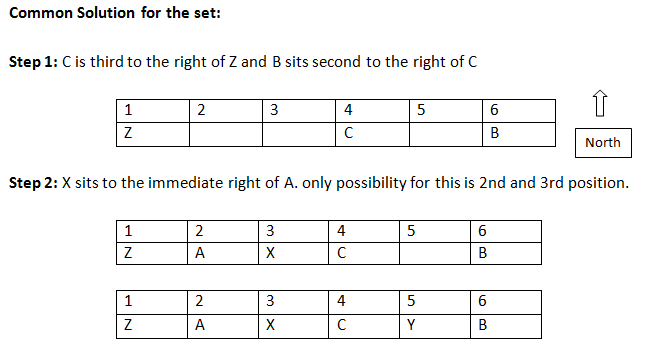### Logical Reasoning clat: Logical Reasoning clat type questions that help in improving your analytical abilities for the clat exam

Logical Reasoning clat type questions with clear solutions are helpful in preparing for the exam. Hence, first attempting the set and then going through the solution helps in confirming that you follow the best possible approach. Logical Reasoning clat type question sets cover some of the commonly asked topics such as direction, arrangement and position based problems. To improve your performance in the analytical section of clat exam, solve questions on a variety of topics using the reference of past year Logical Reasoning clat questions.

Directions for the question set:
Study the following information to answer the given questions:
A, B, C, X, Y, Z are seated in a straight line facing North. C is third to the right of Z and B sits second to the right of C. X sits to the immediate right of A.

Question 1: Which of the following represents the pairs of persons sitting exactly in the middle of the line?
(a) XB
(b) ZB
(c) BX
(d) XC
(e) XY

Question 2: What is X’s position with respect to Z?
(a) Immediate right of Z
(b) Second to the left
(c) Third to the right
(d) Second to the right
(e) None of these

Question 3: Four of the following five are alike in a certain way based on their seating positions in the above arrangement and so form a group. Which is the one that does not belong to the group?
(a) ZA
(b) XC
(c) CY
(d) YB
(e) XA

Question 4: How many persons are seated between A and C ?
(a) One
(b) Two
(c) Three
(d) Four
(e) None of these

### Answers and Explanations: Click the down arrow to expandAnswer 1: (d) XC is in the middle of the arrangement. Thus, the correct option is (d).

Answer 2: (d) X is 2nd to the right of Z. Thus, the correct option is (d).

Answer 3: (e) In all the options first person is sitting to the left of the second person. This arrangement is not followed in option (e). Thus, the correct option is (e).

Answer 4: (a) X is the person sitting between A and C. Thus, the correct option is (a).

Extra tips for Logical Reasoning clat :
• Attempt the questions that you are sure about first. This saves you plenty of time to try out the ones that you uncertain about and hence the ones that may take more time.
• Go through past year Logical Reasoning clat type questions from various topics to get a strong hold on some of the commonly tested topics of CLAT exam.